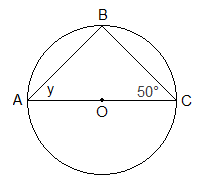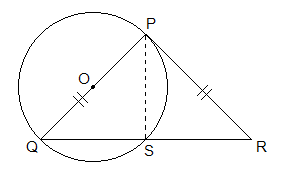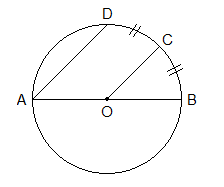# Circle Theorems on Central Angles and Inscribed Angles## Central Angles and Inscribed Angles

The angle formed at the centre of a circle is called a central angle and the angle formed at the circumference of a circle is called a circumference angle or inscribed angle.

********************

10 Math Problems officially announces the release of Quick Math Solver, an Android App on the Google Play Store for students around the world.

********************To understand and prove the circle theorems on central angles and inscribed angles, we use the following axioms on arcs and angles subtended by them:

1.   Degree measurement of a full circle arc is equivalent to 360°.

2.   Central angle of a circle is equal to the degree measurement of It's opposite arc.

3.   Inscribed angle of a circle is equal to half of the degree measurement of its opposite arc.### Theorems:

1.   Central angle of a circle is double of inscribed angle standing on the same arc.

2.   The angles at the circumference of a circle standing on the same arc are equal.

3.   Inscribed angle on a semi-circle is a right angle.

### Proofs:

1.   Central angle of a circle is double of inscribed angle standing on the same arc.Given: O is the centre of a circle. AOB is a central angle and ACB is an inscribed angle standing on the same arc AB.

To Prove: AOB = 2ACB

Proof:

Statements                 Reasons

1.   AOB = Arc AB ----> Central angle is equal to its opposite arc.

2.   ACB = ½ Arc AB ----> Inscribed angle is equal to half of its opposite arc.

3.   2ACB = Arc AB -----> From statements 2.

4.   AOB = 2ACB -----> From statements 1 and 2.

Proved.

2.   The angles at the circumference of a circle standing on the same arc are equal.Given: O is the centre of a circle. ABC and ADC are the inscribed angles standing on same arc AC.

Proof:

Statements                 Reasons

1.   ABC = ½ Arc AC ----> Inscribed angle is equal to the half of It's opposite arc.

2.   ADC = ½ Arc AC ----> Inscribed angle is equal to the half of its opposite arc.

3.   AOB = 2ACB -----> From statements 1 and 2.

Proved.

3.   Inscribed angle on a semi-circle is a right angle.Given: O is the centre of a circle. AB is a diameter.

To Prove: ACB = 90°

Proof:

Statements             Reasons

1.   AOB = 180° ------> Being AOB a straight angle.

2.   ACB = ½ AOB ---> Inscribed angle is equal to the half of its opposite arc.

3.   ACB = ½ × 180° ---> From statements 1 and 2.

4.   ACB = 90° -------> From statement 3.

Proved.

### Worked Out Examples:

Example 1: Find the value of x and y in the given figure.Solution:

From the figure,

OCA = OAC = 50° [Being  OA = OC, Radii of same circle]

y + 50° + 50° = 180° [Sum of  the angels of DOAC]

or,    y + 100° = 180°

or,    y = 180° - 100°

or,    y = 80°

Now,

x = ½ of y [Inscribed angle is half of central angle]

= ½ × 80°

= 40°

Example 2: Find the value of y in the given figure.Solution:

From the figure,

ABC = 90° [An angle formed on semi-circle]

y + 50°  + 90° = 180° [Sum of the angles of DABC]

or,    y + 140° = 180°

or,    y = 180° - 140°

= 40°

Example 3: Find the value of z in the given figure.Solution:

From the figure,

Reflex AOC = 2ABC [Central angle is double of inscribed angle on the same arc]

= 2 × 130°

= 260°

Now,

z + reflex AOC = 360° [Angles at one complete rotation]

or,    z + 260° = 360°

or,    z = 360° - 260°

or,    z = 100°

Example 4: What is the size of CBX in the given figure?Solution:

From the figure,

ADB = 32° and BXC = 85°

ACB = ADB = 32° [Inscribed angles on same arc]

Now,

CBX + ACB + BXC = 180° [Sum of angles of DBXC]

or,    CBX + 32° + 85° = 180°

or,    CBX + 117°= 180°

or,    CBX = 180° - 117°

or,    CBX = 63°

Example 5: In the given diagram, PQR is an isosceles triangle with PQ = PR and a circle is drawn with PQ as a diameter. Prove that QS = RS.Solution:

Given: In ΔPQR, PQ = PR. O is centre and PQ is a diameter of circle.

To Prove: QS = RS.

Construction: Points P and S are joined.

Proof:

Statements        Reasons

1.   PSQ = 90° ----> Being PSQ is in semi-circle with diameter PQ

2.   In ΔPSQ and ΔPSR

i.    PSQ = PSR (R) ----> Each of 90° (Statement 1)

ii.    PQ = PR (H) -----> By given

iii.    PS = PS (S) -----> Common side.

3.   ΔPSQ  ΔPSR -------> By RHS axiom

4.   QS = RS ------> Corresponding sides of  triangles.

Proved.

Example 6: In the given figure, O is the centre of the circle, PQ the diameter and AOPQ, prove that PBR = PAO.Solution:

Given: PQ is the diameter of a circle with centre at O and APPQ.

To Prove: PBR = PAO.

Construction: Points R and Q are joined.

Proof:

Statements       Reasons

1.   PRQ = 90° ----> Being PRQ is in semi-circle with diameter PQ

2.   In ΔPQR and ΔPAO

a)   PRQ = PAO ----> Being APPQ, and from statement 1.

b)   QPR = APO ----> Common angle.

c)   PQR PAO ----> Remaining angles.

3.   PQR = PBR -----> Being inscribed angles on same arc PR.

4.   PBR = PAO ----> From statements 1(c) and 3.

Proved.

Example 7: In the given figure, O is the centre of the circle, AB the diameter and arc BC = arc CD. Prove that AD || OC.Solution:

Given: O is the centre of circle. AB is the diameter. Arc BC = arc CD

Proof:

Statements             Reasons

1.   BAD = ½ of arc BCD ---> Inscribed angle is equal to the half of its opposite arc

2.   BAD = ½ of arc (BC + CD) ---> whole part axiom from statement 1

3.   BAD = ½ of arc (BC + BC) ---> Being arc BC = arc CD (given).

4.   BAD = ½ × arc 2BC ---> From statements 3.

5.   BAD = arc BC ---> From statement 4.

6.   BOC = arc BC ---> Central angle is equal to its opposite arc.

7.   BAD = BOC ---> From statements 5 and 6.

8.   AD // OC ---> Being corresponding angles equal (statement 7)

Proved.

#### Do You Have Any Questions regarding the circle theorems?

You can ask your questions or problems here in the comment section below.

#### 1 comment:

1.